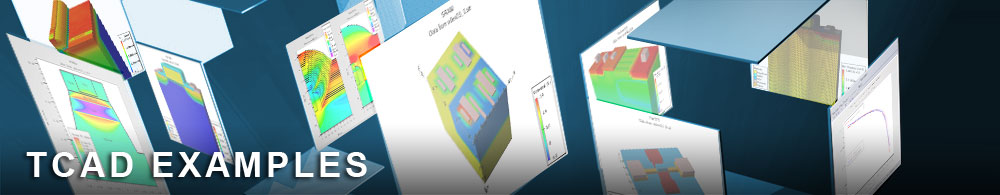LDMOS Breakdown using Ionization Integrals

## powerex08.in : LDMOS Breakdown using Ionization Integrals

Requires: SSuprem 4/S-Pisces
Minimum Versions: Athena 5.22.3.R, Atlas 5.28.1.R

This examples demonstrates a technique used to quickly estimate the breakdown voltage of any device. It uses the same LDMOS power device process simulation and structure as in the previous example.

Rather than perform a full breakdown analysis by solving for impact ionization using a two carrier approach, this example uses ionization integrals. Ionization integrals are based solely on the electric field strength so zero carrier solutions can be done. The zero carrier option is specified using carriers=0 . Although impact ionization is not solved directly, the impact model is used in the postprocessing analysis. For this it is necessary to specify impact selb .

The ionization integrals are calculated along electric field lines. Electric field lines are specified during the solution by parameters on the solve statement. The parameter deltav specifies an offset between the applied voltage and the voltage at which the electric field lines will start. The lines should not start exactly at a contact since the electric field is often zero at the contact. The lratio parameter specifies the ratio of spacing between adjacent field lines. A value less than unity means the lines occur more the left, greater than unity they occur more to the right. In this example the drain is on the right of the structure. So the lines should be more towards the high field area on the left side of the drain. Hence lratio is less than one. The locations of the electric field lines can be saved into the solution files. However the output statement must be used to specify the electric field line parameters as in the solve statement. The final plot shows the electric field and the electric field lines at the breakdown point.

The solve sequence in this example uses assigned variables from the extract statement to closely define the breakdown. The first solve statement ramps the drain voltage in 5V steps until the ionization integrals exceed one. This means the breakdown is between this voltage and 5V less than this voltage. The extract sequence steps the voltage back 5V. The 1V steps are taken until the breakdown condition is reached and the analysis is repeated. After this, 0.1V steps are used to give a breakdown number to within 0.1V.

Users should note that using this method to extract the breakdown voltage is only approximate. The algorithm does not consider any current and hence usually overestimates the actual breakdown voltage. The advantage over the method in the previous example is speed.

To load and run this example, select the Load button in DeckBuild > Examples. This will copy the input file and any support files to your current working directory. Select the Run button in DeckBuild to execute the example.